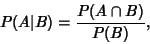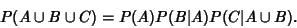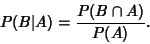## Conditional Probability

The conditional probability ofgiven thathas occurred, denoted, equals(1)

which can be proven directly using a Venn Diagram. Multiplying through, this becomes(2)

which can be generalized to(3)

Rearranging (1) gives(4)

Solving (4) forand plugging in to (1) gives(5)